The new Marimekko chart is similar to a Full stacked column chart but it is two dimensional as also the column width varies based on the data in the chart. As you can display two dimensions in one chart, for example which brand each respondent is using split by age group, you can quickly identify large segments.

Here we see a Marimekko chart, in this example the chart displays Age groups splits by Brand usage.In general, the setup is similar to setting up other chart types but with some limitations:

• The Marimekko chart can only be used for quantitative data which means that it is supported when calculating percentage shares, counts and sums only

• Significance testing and benchmark calculations are not applied to the aggregated values (the values displayed above the stack)

• Percentiles and mean value series cannot be applied to a Marimekko chart

• Sorting by value in a Marimekko cannot be based on the aggregated values

• Controls for hiding date gaps, compare series without data etc. are hidden and always on when a Marimekko is used, as a Marimekko chart cannot show empty data series

• A Marimekko chart can only consist of one series so multiple series cannot be used when Marimekko is the selected chart type.

To setup a Marimekko add a chart object to your StoryTeller, select Marimekko in the chart selection list and select the desired questions, filters and time settings in the same manner as always.

In the example image below Current operator is the compare filter (positioned in Axis) and the Age group is the question (answers are positioned in the legend).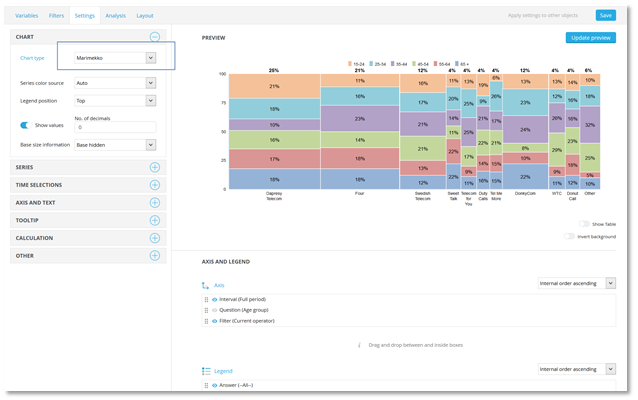The aggregated data values displayed above the stack appear only when “Show value” is enabled, as in the example shown below. The format of the aggregated data values is always the same as the data values displayed inside the column stacks but shown in a bold style and use the same number of decimals and same suffix/prefix as the stacked data values.

Here we see a Marimekko chart that displays data values for the aggregated result, these values are only shown if “Show value” is enabled.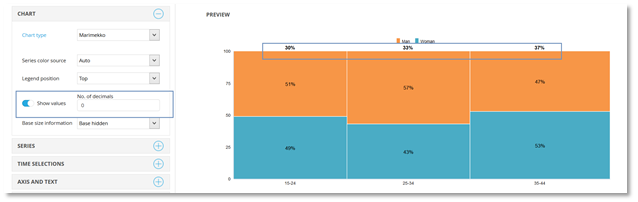Note: depending on the size of the chart and the number of axis objects there is a chance that labels are displayed on top of each other due to lack of space, see example of that in the first image below. The issue can be avoided by setting a fixed label wrapping as shown in the second image below or by rotating the labels as shown in the third image below.

Here we see an example of labels displayed on top of each other.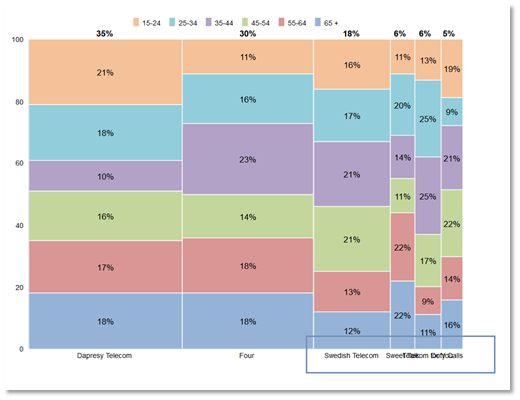Here we see how Fixed wrapping of labels have been applied to the axes labels.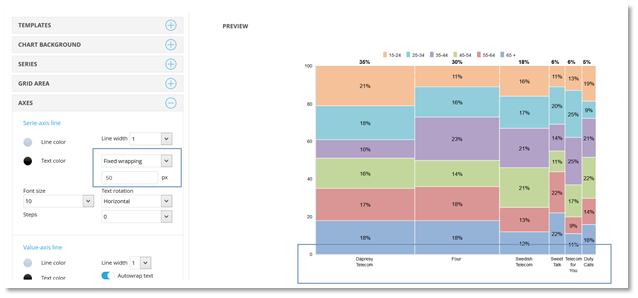Here we see how the axes labels have been rotated.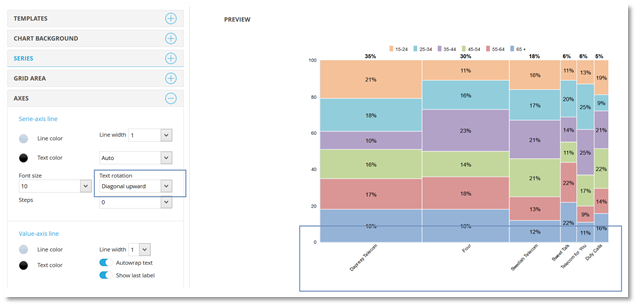The calculation of the stacked result in the Marimekko works in same way as in any other chart type. The aggregated result (displayed on top of the stacked) is calculated based on the counts in the stack in exactly the same way as the aggregated result in a Treemap is calculated. Below we see a basic example. Gender is the selected question and Age the selected compare filter, in the table below the chart you see how the aggregated result was calculated.